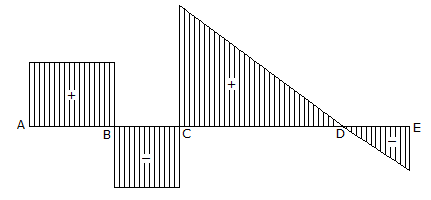# Civil Engineering - UPSC Civil Service Exam Questions

36.

Consider the following statements- with reference to a continuous beam supported at A, C and E for which the shear force diagram is shown in the given figure.There is
1. a concentrated load acting at point B
2. a concentrated load acting at point D
3. a uniformly distributed load acting on the portion CE.
Of these statements:A. 1, 2 and 3 are correct B. 1 and 2 are correct C. 2 and 3 are correct D. 1 and 3 are correct

Explanation:

No answer description available for this question. Let us discuss.

37.

A bullet of mass 0.01 kg moving with a velocity of 401 m/s strikes a block of mass 4 kg which is free to move in the direction of the bullet, and gets embedded in it. The overall loss of kinetic energy is

 A. 80.2 Nm B. 401 Nm C. 802 Nm D. 1604 Nm

Explanation:

No answer description available for this question. Let us discuss.

38.

A Treadle bar is used for

 A. Interlocking points and signal B. Setting points and crossings C. Setting marshalling yard signals D. track maintenance

Explanation:

No answer description available for this question. Let us discuss.

39.

A vehicle is moving up an incline when the driver applies its brakes and the vehicle retards at 0.5 m/s2. Then according to D'Alembert's principle

 A. inertia force will be directed in the direction of motion B. inertia force will be directed opposite to the direction of motion C. magnitude of the inertia force will depend on the angle of inclination D. magnitude of the inertia force will depend on coefficient of friction between the wheels and the road surface

Explanation:

No answer description available for this question. Let us discuss.

40.

Consider the following statements :
Of these statements :

 A. Both A and R are true and R is the correct explanation of A B. Both A and R true but R is not a correct explanation of A C. A is true but R is false D. A is false but R is true